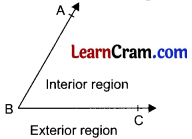# DAV Class 6 Maths Chapter 10 Worksheet 1 Solutions

The DAV Maths Book Class 6 Solutions and DAV Class 6 Maths Chapter 10 Worksheet 1 Solutions of Angles offer comprehensive answers to textbook questions.

## DAV Class 6 Maths Ch 10 WS 1 Solutions

Question 1.
Name the following angles: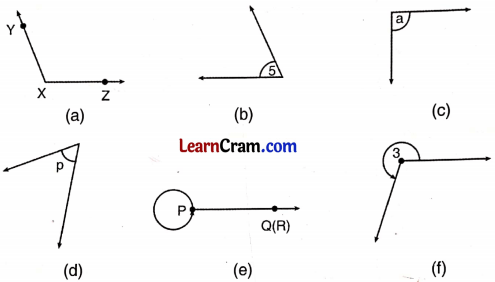(a) ∠YXZ
(b) ∠5
(c) ∠a
(d) ∠P
(e) ∠RPQ
(f) ∠3

Question 2.
Draw the following angles as per their types (use scale and pencil only):
(a) ∠LMN (Reflex angle)(b) ∠y (Obtuse angle)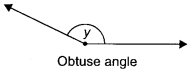(c) ∠XYZ (complete angle)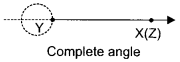(d) ∠3 (Reflex angle)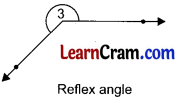Question 3.
Arrange the measure of the following angles in ascending order. Obtuse angle, Straight angle, Zero angle, Right angle, Reflex angle, Complete angle, Acute angle.
Zero angle, Acute angle, Right angle, Obtuse angle, Straight angle, Reflex angle, Complete angle.Question 4.
Look at the figure and answer the questions: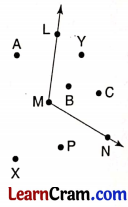(a) Name the points in the interior of ∠LMN
(b) Name the points which lie in the angular region of ∠LMN
(c) Name the points which lie in the exterior of ∠LMN.
(a) Points B, Y and C are in the interior of ∠LMN.
(b) Points L, M, N, B, Y and C are in the angular region.
(c) Points A, X and P lie in the exterior of ∠LMN.

Question 5.
Write another name for the following angles in the figure: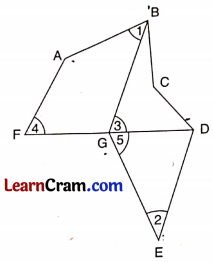(a) ∠1
(b) ∠AFG
(c) ∠2
(d) ∠5
(e) ∠BGD
(a) ∠1 = ∠ABG
(b) ∠AFG = ∠4
(c) ∠2 = ∠GED
(d) ∠5 = ∠EGD
(e) ∠BGD = ∠3

Question 6.
Look at the figure and answer the following questions:(a) Is the point P in the exterior of ∠SOR?
(b) Is the point R in the interior of ∠SOR?
(c) Is the point Q in the interior of ∠ROP?
(d) Is the point S in the exterior of ∠POQ?
(e) Name the point which is in the interior of ∠POR but in the exterior of ∠SOR.
(a) Yes, it is in the exterior of ∠SOR.
(b) No, R is not in the interior of ∠SOR.
(c) Yes, Q is in the interior of ∠ROP.
(d) Yes, S is in the exterior of ∠POQ.
(e) Q is in the interior of ∠POR points P and Q are in the exterior of ∠SOR.Question 7.
Write the kind of angle formed between the following directions:
(a) North and South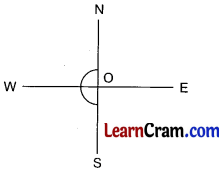∠NOS = 180°
So, it is a straight angle.

(b) North and North-West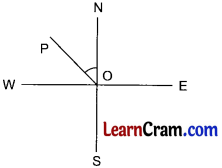∠NOP = 45°
∠NOP is an acute angle

(c) South and East∠SOE = 90°
So, it is right angle.

(d) East and West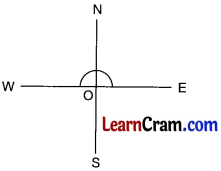∠EOW = 180°
So, it is a straight angle.

Question 8.
Fill in the following blanks:
(a) A reflex angle measures more than _________ and less than _________.
180°, 360°

(b) One complete angle = _________ right angles.
4

(c) The minute hand turns through _________ degrees from the digit 2 to 11 on a clock.
270

(d) An angle of measure 345.5° is a _________ angle.
Reflex

### DAV Class 6 Maths Chapter 10 Worksheet 1 Notes

Look at the figure, B is the vertex of ∠ABC, BA and BC are the arms of ∠ABC. Unit of an angle is ‘degree’. Angle is measured by ‘protractor’.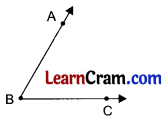Types of Angles
1. Acute angle: The angle whose measure is less than 90° is called an acute angle.
2. Obtuse angle: The angle whose measure is more than 90° but less than 180° is called obtuse angle.
3. Right angle: The angle whose measure is equal to 90° is called right angle.4. Straight angle: The angle whose measure is equal to 180° is called straight angle.
5. Complete angle: The angle whose measure is 360° is called complete angle.
6. Reflex angle: The angle whose measure is more than 180° but less than 360° is called reflex angle.7. Zero angle: The angle whose measure is equal to O’ is called zero angle.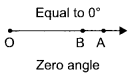Interior and exterior of angles:
The region falling between the arms of the angle is called the interior of the angle and the region which does not fall between the arms of the angle is called exterior of the angle.